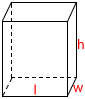# Rectangular Prism Calculator

In geometry, a rectangular prism, known by a number of names including cuboid, is a convex polyhedron bounded by six quadrilateral faces, whose polyhedral graph is the same as that of a cube.Length (l): Width (w): Height (h):